# DAV Class 8 Science Chapter 10 Question Answer – Refraction and Dispersion of Light

The DAV Class 8 Science Book Solutions and DAV Class 8 Science Chapter 10 Question Answer – Refraction and Dispersion of Light are essential study tools for DAV public school students in Class 8.

## DAV Class 8 Science Ch 10 Question Answer – Refraction and Dispersion of Light

### DAV Class 8 Science Ch 10 Solutions – Refraction and Dispersion of Light

Something To Know

A. Fill in the blanks:

Question 1.
A ray of light, passing from one medium to another, does not bend its path if its angle of incidence equals ________ .
90 degree

Question 2.
The more is the optical density of a given medium, the ________ is the speed of light through it.
less

Question 3.
The phenomenon of splitting of white light into seven colours is known as the phenomenon of ________ .
dispersion of light

Question 4.
The point, on the principal axis of a concave lens, from where a beam of incident parallel ray appears to diverge is called the ________ of the concave lens.
focus

Question 5.
An object should be placed at the ________ point so that a convex lens forms its real and inverted image of the same size.
2FB. State True or False for the following statements:

1. The basic cause of refraction is the change in the speed of light as it goes from one medium to another.
True

2. In an optically denser medium, the speed of light is more than the speed of light in vacuum.
False

3. An (obliquely) incident ray always bends away from the normal when it passes from one transparent medium into another.
False

4. A coin, kept at the bottom of an empty dry cup, appears to rise up when some water is poured into the cup.
True

5. When white light is dispersed by a glass prism, the yellow colour in it bends more than the blue colour.
FalseC. Tick (v) the correct option:

Question 1.
Which of the following conditions is not necessary for a change in the direction of propagation when a light ray goes from one medium to another?
(a) The light ray must be incident at an angle greater than 0° with respect to the normal at its point of incidence.
(b) The two media, both must be transparent.
(c) The two media must have different refractive indices.
(d) The incident light rays must always have only the blue colour.
(d) The incident light rays must always have only the blue colour.

Question 2.
Which of the following diagrams correctly shows the “blending of a light ray’ as it goes from an optically denser medium into an optically rarer medium?Question 3.
The angle, between the incident ray and the emergent ray, for a rectangular glass slab is-
(a) 0°
(b) 90°
(c) An obtuse angle
(d) A non-zero acute angle
(a) 0°; because emergent ray is parallel to incident ray

Question 4.
Which of the following figures correctly represents the passage of white light through a glass prism?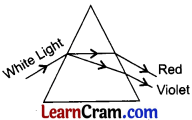Question 5.
An incident ray, passing through the optical centre of a concave lens, after refraction through it, will-
(a) move parallel to its principal axis
(b) pass through its principal focus
(c) go undeviated
(d) pass through the 2F point
go undeviated

Question 6.
When an object approaches a convex lens, from infinity towards its focus, the image formed by it-
(a) keeps shifting away from the lens
(b) keeps shifting towards the lens
(c) shifts first towards, and then away from the lens
(d) shifts first away, and then towards the lens
(a) keeps shifting away from the lens

Question 7.
Tanya’s grandmother needs a lens to read small letters in her book. For this, she should use
(a) a convex lens (of focal length F), kept at a distance between F and 2F from the book.
(b) a concave lens, kept at a distance less than its focal length from the book.
(c) a concave lens, kept at a distance between F and 2F, from the book.
(d) a convex lens, kept at a distance less than its focal length from the book.
(d) a convex lens, kept at a distance less than its focal length from the book.D. Answer the following questions in brief:

Question 1.
Light propagates faster through a Medium A than through another Medium B.
(a) Which of the two media has a higher optical density?
Medium B

(b) State the condition under which no change in the direction of propagation of a light ray would take place when it passes from Medium A to Medium B.
When the incident ray makes a right angle at the point of incidence.

Question 2.
Why does a pencil appear bent when it is partially dipped in water?
When light rays coming from water enter the air, they bend away from normal. Due to this, light rays appear to be coming from a different position than the actual position of the pencil. Due to this, a pencil appears to be bent when it is partially dipped in water.

Question 3.
Trace the path of rays in the following ray diagrams.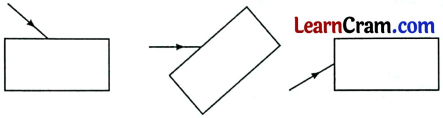Question 4.
When a spherical lens is held towards the Sun and a sharp image is formed on a piece of black paper, a hole gets burnt in the black paper, after some time.
(a) Name the lens used in the above activity.
Convex lens

(b) What name is given to the distance between the spherical lens and the black paper?
Focal length

(c) What is the relative advantage of using a black paper rather than a white paper?
Black colour absorbs most of the energy falling on it, while white colour reflects most of the energy falling on it. So, black paper can bum faster than white paper in this experiment.

Question 5.
What kind of lens would form-
(a) An inverted and magnified image of the object?
Convex lens

(b) An erect and magnified image of the object?
Convex lens

(c) An inverted and diminished image of the object?
Convex lens

(d) An erect and diminished image of the object?
Concave lensQuestion 6.
Draw labeled diagrams to illustrate the differences between the virtual images formed by using
(a) a concave lens
(b) a convex lens.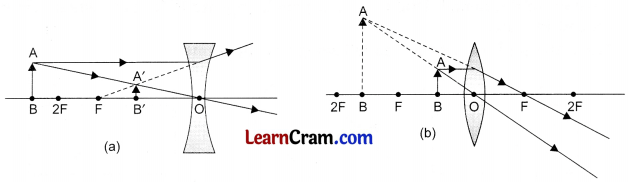Question 1.
A coin is placed at the bottom of a clear glass vessel. An observer moves herself to a position where the coin goes just out of sight of her eyes. Keeping her eyes in that position, she asks her friend to pour some water, gradually into the glass vessel.
(a) What would she observe?
Once water is filled in the vessel, the coin becomes visible to the observer.

(b) Name the phenomenon involved in this observation.
Refraction of light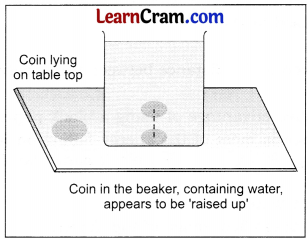Question 2.
In the following figures, identify the light ray that would be correct emergent/ refracted light ray in each case. Give reason for your answer in each case.In case of Fig (a), P is the emergent ray. This can be shown by following figure.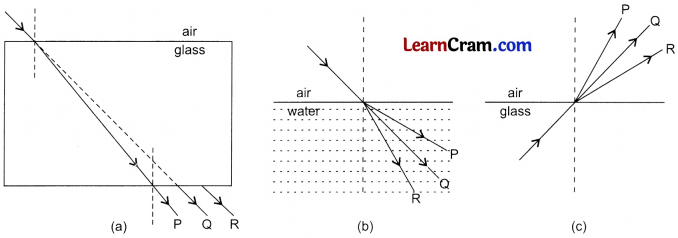In case of figure (b) R is the emergent ray because light ray bends towards the normal when it enters a denser medium from a rarer medium.
In case of fig (c), R is the emergent ray because light ray bends away from the normal when it enters a rarer medium from a denser medium.

Question 3.
White light is made to fall on one face of a glass prism. Draw a ray diagram to show the nature of the emergent beam.Question 4.
With the help of a suitable diagram, explain the meaning of terms:
(a) Centres of curvature
(b) Optical centre
(c) Focus
Centre of curvature: A lens is generally made up of two curved surfaces. These curved surfaces are parts of spheres. Centres of these spheres are called the centre of curvature. Here, Cl and C2 are the centres of curvature.
Optical Centre: The centre inside the lens is called optical centre. A ray of light passing through the optical centre emerges without deviation.Focus: When rays of light parallel to the principal axis, they converge at a point in case of convex lens. In case of a concave lens, they rays appear to diverge from a point. This point is called the focus of the lens.Question 5.
We are given a convex lens of focal length 20 cm. Draw ray diagrams to show the nature, size and position of the image formed when the object is kept at a distance of (a) 55 cm, (b) 35 cm (c) 15 cm
(Note: For drawing ray diagrams, use an appropriate scale.)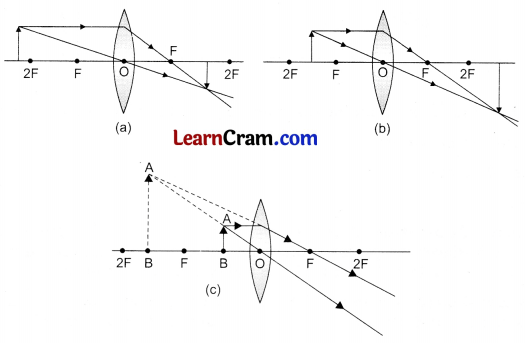Question 6.
We are given a concave lens of focal length 15 cm. Draw a ray diagram to show the nature, size and position of the image formed when the object is kept at a distance of (a) 30 cm (b 15 cm (c) 10 cm
(Note: For drawing ray diagrams, use an appropriate scale.)Question 7.
What type of a lens is used as a magnifying glass? How is the object positioned with respect to this lens? Draw the appropriate ray diagram.
A convex lens is used as a magnifying glass. Object is kept between F and O to get a magnified image.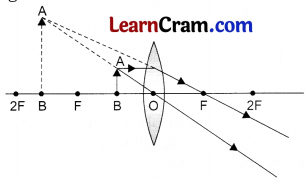Value Based Question

Khushi listened with great attention and interest when her teacher explained the focusing action of a convex lens through the ‘black paper’ experiment. The teacher went on to say that we must have a similar focused and dedicated approach towards the ‘task at hand’ to achieve success in our life.

On reaching home, Khushi shared her teacher’s ideas with her mother. Her mother fully supported her teacher’s suggestion and advised Khushi to do her studies in a focused and concentrated way. She also advised Khushi not to get distracted by messages on social media while she was studying. Khushi promised to do so.

Question 1.
Write the values depicted by (a) Khushi’s teacher (b) Khushi
(a) Khushi’s teacher shows the value of focus and dedication to her task.
(b) Khushi shows honesty and a tendency to share ideas with others.

Question 2.
Draw a diagram showing the focusing action of a convex lens in the black paper experiment mentioned above.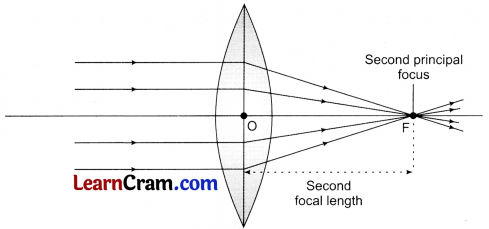Question 3.
Have a group discussion with your friends, on the ‘pros and cons’ of social media in our life.
Do it yourselfSomething To Do

Question 1.
One of the first microscopes invented used a small drop of water as ‘magnifying glass’. The drop was held in a smooth round hole in a sheet of metal or stiff plastic. Design your own magnifying glass using a stiff plastic sheet. Examine a small object (as the text on a page) through the water drop. How does changing the size of the drop affect the magnification of the image? Record your observations.
Do it yourself

Question 2.
Use two appropriate convex lenses and two cylindrical/cardboard tubes to design and optical telescope.
Do it yourself

Question 3.
Take a tub/trough full of water and place a light source (like a water proof pencil torch) inside it. Look at the light beam from above. Now, tilt the pencil torch to change the angle of incidence. Observe carefully what happens? Do you observe any refracted beam when you increase the angle of incidence beyond a certain value?
Do it yourself### DAV Class 8 Science Chapter 10 Solutions – Refraction and Dispersion of Light Important Questions and Answers

I. Fill in the blanks:

1. Glass is optically ________ than water.
denser

2. Air is optically ________ than water.
rarer

3. The speed of light is highest in ________ .
vacuum

4. At least one surface of the lens should be ________ .
spherical

5. A concave lens always makes ________ erect and smaller image of an object.
virtual

Question 1.
How many colours mix together to make white light?
(a) 5
(b) 7
(c) 9
(d) 11
(b) 7

Question 2.
Which phenomena is used in the formation of the rainbow?
(a) Reflection of light
(b) Refraction of light
(c) Dispersion of light
(d) None of these
(c) Dispersion of light

Question 3.
Which of the object is used for the dispersion of light?
(a) Glass slab
(b) Prism
(c) Convex lens
(d) Concave lens
(b) Prism

Question 4.
Which of these is used as a magnifying glass?
(a) Glass slab
(b) Prism
(c) Convex lens
(d) Concave lens
(c) Convex lensQuestion 5.
Which type of image do we see when we are reading through a magnifying glass?
(a) Real and inverted
(b) Real and erect
(c) Virtual and inverted
(d) Virtual and erect
(d) Virtual and erect

Question 1.
Define refraction of light.
When a ray of light travels from one transparent medium to another transparent medium, it changes its direction of propagation. This phenomenon is called refraction of light.

Question 2.
What do you understand by refractive index? Explain.
Refractive Index: The ratio of speed of light; in a given pair of media gives the value of relative refractive index for the given pair of media.

Relative refractive index of medium 2 with respect to medium 1
²1μ = $$\frac{\text { Speed of light in medium 1 }}{\text { Speed of light in medium 2 }}=\frac{v_1}{v_2}$$
The refractive index or absolute refractive index of a medium (m), denoted by (μm) is given as follows:
μm = $$\frac{\text { Speed of light in vacuum C }}{\text { Speed of light in the given medium }}=\frac{C}{v_m}$$

Question 3.
State the various rules of refraction.
When a ray of light travels (obliquely) from an optically rarer medium to an optically denser medium, it bends towards the normal.
When a ray of light travels (obliquely) from an optically denser medium to an optically rarer medium, it bends away from the normal.

Question 4.
What is dispersion of white light? Why does it happen?
When white light passes through a glass prism, it segregates into its component colours. This phenomenon is called dispersion of light. The speed seven of light of different colours are different. Different colours deviate differently because of the difference in speed. This is the reason of dispersion of white light when it passes through a glass prism.Question 5.
How is a rainbow formed?